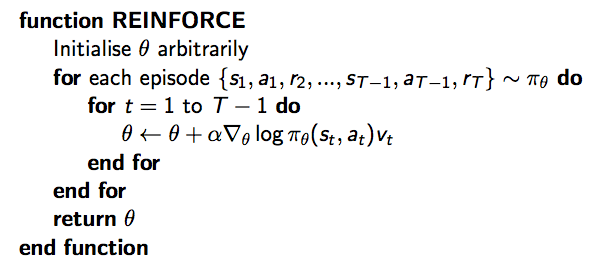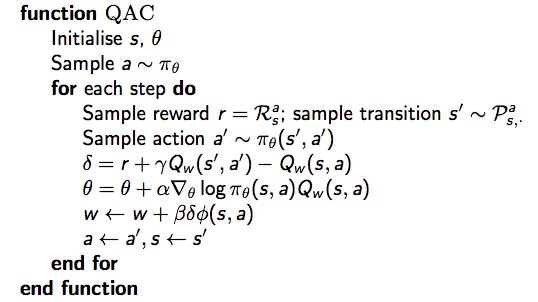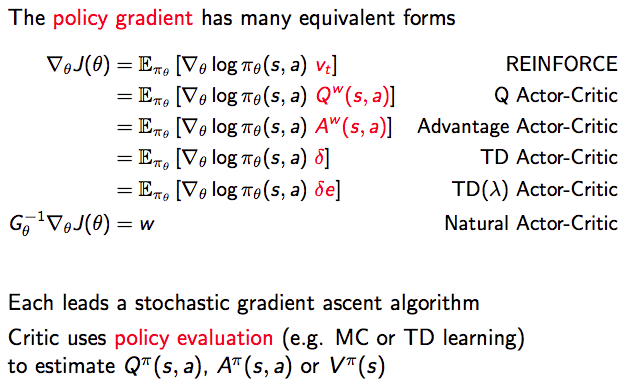# [Reinforcement Learning] Policy Gradient Methods

$V_{\theta}(s)\approx V^{\pi}(s) \\ Q_{\theta}(s)\approx Q^{\pi}(s, a)$

$\pi_{\theta}(s, a) = P[a | s, \theta]$

# Value-Based vs. Policy-Based RL

Value-Based：

• 学习价值函数
• Implicit policy，比如 $\epsilon$-greedy

Policy-Based：

• 没有价值函数
• 直接学习策略

Actor-Critic：

• 学习价值函数
• 学习策略

![](https://img2018.cnblogs.com/blog/764050/201811/764050-20181102094901268-512503979.png)

• 收敛性更好
• 对于具有高维或者连续动作空间的问题更加有效
• 可以学习随机策略

• 绝大多数情况下收敛到局部最优点，而非全局最优
• 评估一个策略一般情况下低效且存在较高的方差

# Policy Search

## Policy Objective Functions

• 对于episode 任务来说，我们可以使用start value：

$J_1(\theta)=V^{\pi_{\theta}}(s_1)=E_{\pi_{\theta}}[v_1]$

• 对于连续性任务来说，我们可以使用 average value：

$J_{avV}(\theta)=\sum_{s}d^{\pi_{\theta}}(s)V^{\pi_{\theta}}(s)$

$J_{avR}(\theta)=\sum_{s}d^{\pi_{\theta}}(s)\sum_{a}\pi_{\theta}(s, a)R_s^a$

• 爬山算法
• 模拟退火
• 进化算法
• ...

• 梯度下降
• 共轭梯度
• 拟牛顿法
• ...

# 策略梯度定理

$\triangledown_{\theta}\pi_{\theta}(s, a) = \triangledown_{\theta}\pi_{\theta}(s, a) \frac{\triangledown_{\theta}\pi_{\theta}(s, a)}{\pi_{\theta}(s, a)}=\pi_{\theta}(s, a)\triangledown_{\theta}\log\pi_{\theta}(s, a)$

$J(\theta)=E_{\pi_{\theta}}[r] = \sum_{s\in S}d(s)\sum_{a\in A}\pi_{\theta}(s, a)R_{s, a}\\ \triangledown_{\theta}J(\theta) = \color{Red}{\sum_{s\in S}d(s)\sum_{a\in A}\pi_{\theta}(s, a)}\triangledown_{\theta}\log\pi_{\theta}(s, a)R_{s, a}=E_{\pi_{\theta}}[\triangledown_{\theta}\log\pi_{\theta}(s, a)r]$

$\triangledown_{\theta}J(\theta) = E_{\pi_{\theta}}[\triangledown_{\theta}\log\pi_{\theta}(s, a)Q^{\pi_{\theta}}(s, a)]$

$\triangledown_{\theta}J(\theta) = E_{\pi_{\theta}}[\triangledown_{\theta}\log\pi_{\theta}(s, a)Q^{\pi_{\theta}}(s, a)]$

# 蒙特卡洛策略梯度算法（REINFORCE）

Monte-Carlo策略梯度算法，即REINFORCE：

• 通过采样episode来更新参数：；
• 使用随机梯度上升法更新参数；
• 使用return $v_t$ 作为 $Q^{\pi_{\theta}}(s_t, a_t)$ 的无偏估计

$\Delta\theta_t = \alpha \triangledown_{\theta}\log\pi_{\theta}(s_t, a_t)v_t$，具体如下：# Actir-Critic 策略梯度算法

Monte-Carlo策略梯度的方差较高，因此放弃用return来估计行动-价值函数Q，而是使用 critic 来估计Q：

$Q_w(s, a)\approx Q^{\pi_{\theta}}(s, a)$

• Critic：更新动作价值函数参数 $w$
• Actor： 朝着 Critic 方向更新策略参数 $\theta$

Actor-Critic 算法是一个近似的策略梯度算法：

$\triangledown_\theta J(\theta)\approx E_{\pi_{\theta}}[\triangledown_{\theta}\log \pi_{\theta}(s, a)Q_w(s, a)]\\ \Delta\theta = \alpha\triangledown_\theta\log\pi_{\theta}(s,a)Q_w(s,a)$

Critic 本质就是在进行策略评估：How good is policy $\pi_{\theta}$ for current parameters $\theta$.• 价值函数的估计值没有和策略相违背，即：$\triangledown_w Q_w(s,a) = \triangledown_\theta\log\pi_{\theta}(s,a)$
• 价值函数的参数w能够最小化误差，即：$\epsilon = E_{\pi_{\theta}}[(Q^{\pi_{\theta}}(s, a) - Q_w(s,a))^2]$

## 优势函数

\begin{align} E_{\pi_{\theta}}[\triangledown_\theta\log\pi_{\theta}(s,a)B(s)] &=\sum_{s\in S}d^{\pi_{\theta}}(s)\sum_a \triangledown_\theta\pi_{\theta}(s, a)B(s)\\ &=\sum_{s\in S}d^{\pi_{\theta}}(s)B(s)\triangledown_\theta\sum_{a\in A}\pi_{\theta}(s,a )\\ &= 0 \end{align}

$A^{\pi_{\theta}}(s,a)=Q^{\pi_{\theta}}(s,a)-V^{\pi_{\theta}}(s)\\ \triangledown_\theta J(\theta)=E_{\pi_{\theta}}[\triangledown_\theta\log\pi_{\theta}(s,a)A^{\pi_{\theta}}(s,a)]$

$V^{\pi_{\theta}}(s)$ 是真实的价值函数，TD算法利用bellman方程来逼近真实值，误差为 $\delta^{\pi_{\theta}}=r+\gamma V^{\pi_{\theta}}(s') - V^{\pi_{\theta}}(s)$。该误差是优势函数的无偏估计。因此我们可以使用该误差计算策略梯度：

$\triangledown_\theta J(\theta)=E_{\pi_{\theta}}[\triangledown_\theta\log\pi_{\theta}(s,a)\delta^{\pi_{\theta}}]$# Reference

posted @ 2018-11-02 09:52  Poll的笔记  阅读(...)  评论(...编辑  收藏